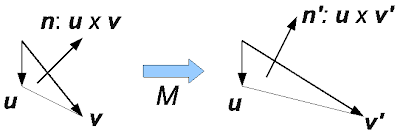## Normal vector as a perpendicular vector of the surface tangent vectors

Normal vector has the same direction to the cross product of two tangent vectors of a surface.  Figure 2 shows the tangent vectors are correctly transformed by the matrix M that magnifies only x direction. However, their cross product is not necessary to the same as the transformation of normal by the matrix M.Figure 2. The normal vector n is a cross product of tangent vectors u and v. Tangent vectors are linear to M, but not for the normal vector.
In short, tangent vectors u, v can be transformed by M, but their cross product is not. In general,
Are you convinced this is the reason distinguishing a usual vector and a normal vector? If you think about the x component of the cross product, uy vz - uz vy, this is not linear. Therefore, a linear transformation cannot transform this. This is my first explanation.